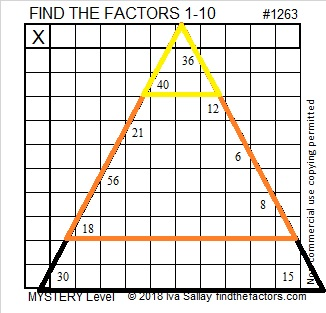# 1263 Candy Corn Mystery

Candy Corn is a soft traditional Halloween candy. I hope this puzzle is a sweet treat for you to solve! Just write the numbers from one to 10 in the first column and the top row so that the puzzle becomes a sort of multiplication table with the given clues becoming the products of the factors you write.Print the puzzles or type the solution in this excel file: 10-factors-1259-1270

Now I’ll write a little bit about the number 1263:

• 1263 is a composite number.
• Prime factorization: 1263 = 3 × 421
• The exponents in the prime factorization are 1 and 1. Adding one to each and multiplying we get (1 + 1)(1 + 1) = 2 × 2 = 4. Therefore 1263 has exactly 4 factors.
• Factors of 1263: 1, 3, 421, 1263
• Factor pairs: 1263 = 1 × 1263 or 3 × 421
• 1263 has no square factors that allow its square root to be simplified. √1263 ≈ 35.538711263 is the hypotenuse of a Pythagorean triple:
87-1260-1263 which is 3 times (29-420-421)

This site uses Akismet to reduce spam. Learn how your comment data is processed.